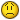Calculating Conditional Standard Deviation?

G

Guest

Hi there,

I have two columns - Industry Code and Market Cap. I want to create a
summary table for average market cap and standard deviation for certain
Industries. However, there are like 20 different industry codes and I didn't
exactly feel like doing it manually for 20 cells. I tried out this formula
first:

{=STDEV((--(\$E\$2:\$E\$296=K17))*\$F\$2:\$F\$296/1000000)}

Using it an array - the Ctrl+Shift+Enter thing. That didn't work out too well.

Cells E2:E296 contained the Industry Code column. Cells F2:F296 contained
the market capitlisations, while K17 was one of the 20 industry codes.

I think the reason why it didn't work (apart from probably wrong usage of
the double negatives on my part) was that it produced zeros for the companies
that I didn't want to include, which could screw up the STDEV calc, since it
includes any zeros in the calculations.

Is there any way around this?

I thought there might be another way by using standard deviation = square
root (expected value of x^2 - (average of x)^2 ) but couldn't really figure
out how to do a conditional squared sum. Is there of a way of doing that?

Iwan J

G

Guest

Nevermind, I figured out a way by using OFFSET.

However, I would still be curious if there was another way of doing this...

T

T. Valko

Try it like this: (array entered)

=STDEV(IF(\$E\$2:\$E\$296=K17,\$F\$2:\$F\$296/1000000))

Biff

G

Guest

It didn't work, unfortunately.T. Valko said:
Try it like this: (array entered)

=STDEV(IF(\$E\$2:\$E\$296=K17,\$F\$2:\$F\$296/1000000))

Biff

T

T. Valko

Hmmm....

Well, it was the corrected equivalent of:
{=STDEV((--(\$E\$2:\$E\$296=K17))*\$F\$2:\$F\$296/1000000)}

You were correct in your assumption about producing 0's and skewing the
result.

Just multiplying: (\$E\$2:\$E\$296=K17)*\$F\$2:\$F\$296/1000000

Will produce the 0's so the double unary in this case was redundant.

The syntax I used takes care of that.

Biff

G

Guest

Please clarify the meaning of "It didn't work". If array entered, the
formula will produce the standard deviation of the values in column F divided
by 1000000 that correspond to values in column E which equal K17. Was the
formula not array entered, or was that standard deviation not what you
wanted?

Another possibility is that there may be issues with the matching to K17.
IF K17 appears to be a number, either it or some of the "matching values" may
be a string instead of a number, an therefore not a match. If K17 is
supposed to be a string, there may be differences in trailing spaces that
prevent a match.

Jerry